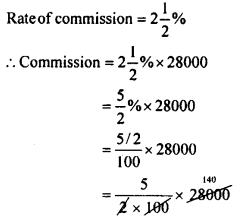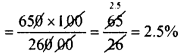# KSEEB Solutions for Class 8 Maths Chapter 9 Commercial Arithmetic Ex 9.4

In this chapter, we provide KSEEB SSLC Class 8 Maths Chapter 9 Commercial Arithmetic Ex 9.4 for English medium students, Which will very helpful for every student in their exams. Students can download the latest KSEEB SSLC Class 8 Maths Chapter 9 Commercial Arithmetic Ex 9.4 pdf, free KSEEB SSLC Class 8 Maths Chapter 9 Commercial Arithmetic Ex 9.4 pdf download. Now you will get step by step solution to each question.

### Karnataka State Syllabus Class 8 Maths Chapter 9 Commercial Arithmetic Ex 9.4

Question 1.
Sindu sells her scooty for Rs. 28,000 through a broker. The rate of brokerage is 2%. Find the commission that the agent gets and the net amount Sindhu gets.
Price of the scooty Rs. 28,000Commission = Rs. 700
The agent gets Rs. 700
Net amount sindhu gets = 28000 – 700 = Rs. 27,300

Question 2.
A share agent sells 2000 shares at Rs. 45 each and gets the commission at the rate of 1.5%. Find the amount the agent gets.
The S.R of 1 share = Rs. 45
The S.P. of 2000 shares = 2000 × 45 = Rs. 90,000
The rate of commission = 1.5%
∴ The commission =$\frac{1.5 \times 90000}{100}$ = Rs. 1350.0
The agent gets Rs. 1350.

Question 3.
A person insures Rs. 26,000 through an insurance agent. If the agent gets Rs. 650 as the commission. find the rate of commission.
For Rs. 26,000 the commission is Rs. 650
For Rs. 100 the commissionRate of commission = 2.5%

Question 4.
A selling agent gets Rs. 10,200 in a month. This includes his monthly salary of Rs. 6000 and 6% commission for the sales. Find the value of the goods he sold.
Commission = 10,200 – 6000 = Rs. 4200
Rate of commission = 6%
∴ If the commission is Rs. 6 then the value of goods = 100
If the commission is Rs. 4200 the value of goods.$\frac{100 \times 4200}{6}$
= 100 x 700 = Rs. 70,000
The value of the goods sold by him = Rs. 70,000

All Chapter KSEEB Solutions For Class 8 maths

—————————————————————————–

All Subject KSEEB Solutions For Class 8

*************************************************

I think you got complete solutions for this chapter. If You have any queries regarding this chapter, please comment on the below section our subject teacher will answer you. We tried our best to give complete solutions so you got good marks in your exam.

If these solutions have helped you, you can also share kseebsolutionsfor.com to your friends.

Best of Luck!!